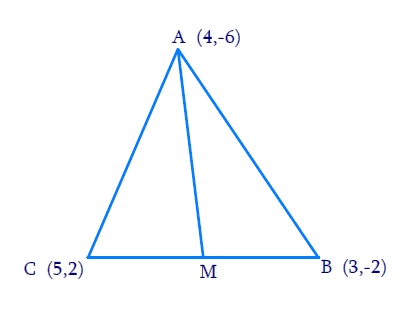# Ex.7.3 Q5 Coordinate Geometry Solution - NCERT Maths Class 10

Go back to  'Ex.7.3'

## Question

You have studied in Class IX that a median of a triangle divides it into two triangles of equal areas. Verify this result for $$\Delta ABC$$ whose vertices are $$A(4, -6),$$ $$B(3, -2)$$ and $$C(5, 2)$$

Video Solution
Coordinate Geometry
Ex 7.3 | Question 5

## Text Solution

Reasoning:

Let $$ABC$$ be any triangle whose vertices are  $$A(x_1, y_1)$$, $$B(x_2, y_2)$$ and $$C(x_3, y_3).$$

Area of a triangle

\begin{align}=\frac{1}{2} \begin{bmatrix} x_1 \left( y_2 - y_3 \right) + \\ x_2 \left( y_3 - y_1 \right) + \\ x_3 \left( y_1 - y_2 \right) \end{bmatrix} \end{align}

What is known?

The $$x$$ and $$y$$ co-ordinates of the vertices of the triangle.

What is unknown?

To verify that a median of a triangle divides it into two triangles of equal areas.

Steps:

From the figure,Given,

• Let the vertices of the triangle be $$A$$$$(4, -6),$$ $$B$$$$(3, -2),$$ and $$C$$$$(5, 2).$$
• Let $$M$$ be the mid-point of side $$BC$$ of $$\Delta ABC.$$

Therefore, $$AM$$ is the median in $$\Delta ABC.$$

Coordinates of point $$M$$

\begin{align}{\text{}}\;{{}} &= \left[ {\frac{{3 + 5}}{2} + \frac{{ - 2 + 2}}{2}} \right] \\ &= [4,0]\end{align}

Area of a triangle

\begin{align}=\frac{1}{2} \begin{bmatrix} x_1 \left( y_2 - y_3 \right) + \\ x_2 \left( y_3 - y_1 \right) + \\ x_3 \left( y_1 - y_2 \right) \end{bmatrix}\;\;\dots(1) \end{align}

By substituting the values of vertices, $$A, B, M$$ in the Equation $$(1)$$

Area of $$\Delta ABM$$

\begin{align} &= \frac{1}{2} \begin{bmatrix} (4)\{ ( - 2) - (0)\} \\ + (3)\{ (0) - ( - 6)\} \\ + (4)\{ ( - 6)\} - ( - 2)\} \end{bmatrix} \\ &= \frac{1}{2}[ - 8 + 18 - 16]\\& = 3\;\text{ Square units }\end{align}

By substituting the values of vertics, $$A,D,C$$ in the Equation $$(1)$$

Area of $$\Delta ABD$$

\begin{align} &= \frac{1}{2} \begin{bmatrix} (4)(( - 2) - (0)) + \3)((0) - ( - 6))+\\((4){ ( - 6)) - ( - 2)} \end{bmatrix} \\&= \frac{1}{2}[- 8 + 18 - 16]\\&= 3{\text{ Square units }}\end{align} However, area cannot be negative. Therefore, area of \(\Delta AMC is $$3\, \rm{square \,units}.$$

Hence, clearly, median $$AM$$ has divided $$\Delta ABC$$ in two triangles of equal areas.

Learn from the best math teachers and top your exams

• Live one on one classroom and doubt clearing
• Practice worksheets in and after class for conceptual clarity
• Personalized curriculum to keep up with school